18.03.2021 г.

Method of projective transformations graph adjustment for improving quality of panorama image stitching

Today we’ll be talking about an approach that can be used to improve the quality of panorama image stitching. There is a widely used method of panorama stitching for images of two-dimensional objects, but it has its disadvantages. That’s why we’d like to propose our own improved version of this method.

Panorama stitching consists in making one composite image using a set of original images (see Figure 1). It is implemented in the following real-life practical tasks:

• Satellite and drone remote sensing of the Earth;
• Stitching of the images collected by a microscope;
• Video stitching;
• Getting extra-high resolution images.

Figure 1 — The original images and the panorama

A general outline of the panorama stitching algorithm can be represented the following way  (see Figure 2). First off, an adequate number of images has to be collected from a video stream. The process of reading images one-by one and selecting the ones with the desired frequency can be performed online.

Figure 2 — The flowchart of the panorama stitching algorithm performance (the algorithm uses keypoints).

Next we’ll have to perform the keypoint detection using a brute force search and calculate their descriptors in these images [2-4]. It’s the keypoints that make it possible to perform geometric matching between two images. The next step is to pair keypoints based on their descriptors. Keep in mind that it’s possible to get false matching results.

After we get two sets of keypoints, we need to find a projective transformation that would transfer the keypoints of one image into matching points of the other image in the best possible way. The RANSAC method can be used to solve this problem . You can find a more in-depth description of this method in the following works [6,7].

In order to find projective transformations between the images, optical flow can be applied as well. It is often used for the panorama image stitching problems .

After the required set of projective transformations is obtained, the technical procedure of image stitching takes place, namely for each pixel of the final panorama image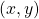and for each channel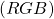a mean intensity value of the pixels with the coordinatesis calculated for all the images containing the pixels with these coordinates.

Shifting of the camera position compared to its previous position in space can be identified using the projective transformation search methods. When working in laboratory conditions, the precision of the calculations of these data is high enough for creating a panorama image of a fixed planar object. In real-world uses, there will be computational errors when calculating the shifting of the camera position with respect to its previous position (measurements errors/interferences/restrictions imposed by the algorithms, etc). And over time cumulative error keeps growing so that despite the acceptable calculation precision of the shift between the consecutive positions, the general panoramic image of the object will have serious discrepancies (see Figure 3).

Figure 3 — Cumulative error

We set ourselves a goal to create a method for projective transformation graph adjustment that can be used to solve the problem of stitching the panorama of fixed planar objects and that will not be prone to error accumulating. Another goal we had while developing this method was to build it in such a way as not to depend on the calculation method of the projective transformation parameters.

One of the following conditions must be fulfilled:

• A fixed pseudorigid object is being surveyed;
• An object that can be characterized as two-dimensional is being surveyed from a large enough distance;
• The requirement is met for all the camera positions during the survey: the lines that connect the points of the image to the camera focus do not match, and it’s true for all the points of the image.

The description of the algorithm for projective transformations graph adjustment

Let’s introduce the concept of the uniform coordinate system. The term uniform coordinate system will be used to define a coordinate system where the same points of the object will have the same coordinates in different images. This requirement can be expressed with a simple formula: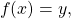Where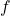is the mapping which is defined for the general part of the images and which transforms the points of the first image into the points of the second image,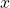is the coordinates of the point in the coordinate system of the first image,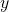is the coordinates of the point in the coordinate system of the second image.

If the mappingcan be correctly continued beyond the point where the images overlap, then it’s possible to supplement the second image with the information from the first one. And as a result, we’ll get a map that is put together out of two or more images like a puzzle.

After we find projective transformations between neighboring images, we’ll have the first stitching that determines a unique position of the images in the uniform coordinate system (see Figure 4).

Figure 4 — Unique position of the image on the map

After the initial image stitching, the graph of projective transformations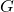is built: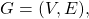Where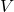is the set of quadruples of points which are the vertices of the projectively transformed images;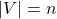,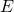is the set of projective transformations between the images;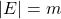.

The line that joins the points is built only if the images form an intersection which is not less than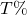in the original stitching (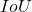— Intersection over Union) (see Figure 5, 6):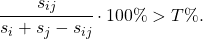Figure 5 — Intersection of the images

The threshold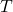is selected depending on the method of the projective transformation search by balancing between the conditionality of the projective transformation search problem and the desired number of edges and cycles in the graph.

Figure 6 — An example of graph building

In the end, the projective transformations graphlooks as follows (see Figure 7):

Figure 7 — The final projective transformations graph

If the graph contains cycles (see Figure 6), then it has redundant data that might contain contradictions. In order to determine what kind of contradictions might arise, let’s review one of the cycles (see Figure 8). Let’s suppose that this cycle consists of the vertices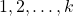. Then we’ll have a set of projective transformations along this cycle: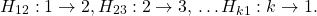Let’s look at the composition of these mappings: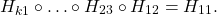Figure 8 — Cycle of the graph

The mapping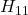is supposed to be an identity mapping. If it’s not, then we can say we got a contradiction. And we’ll call such a cycle inconsistent. This means that now we have a problem of possible inconsistent cycles in the projective transformations graph. When the stitching is performed perfectly, there are not supposed to be any contradictions in the projective transformations graph.

Let’s describe the algorithm for projective transformations graph adjustment, i.e. adjustment of all the cycles of the graph. To minimize cumulative error that occurs when the cycle closes, we are going to use the framework of the SLAM method (Simultaneous Localization and Mapping) .

Let’s review the quadruples of points of general position in each image. If the images are numbered fromto, then the quadruples of points are denoted with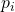, where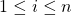. This set of quadruples of points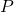uniquely sets the uniform coordinate system since there is only one way to find a projective mapping that transforms one quadruple of points into the next one for any two images.

The least squares method can be used to find the set of quadruples of points that defines the sought-for adjusted graph. We’ll minimize the composite function that is equal to the sum over all the edges from the setof the graph, and for each edge it’s equal to the sum over four points of the values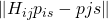. In order to find the solution that minimizes the composite function, we suggest to use the conjugate gradients method.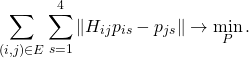Once we get a projective transformation for each image, and these transformations uniquely determine the image positions on the map, we will be able to generate the panorama image.

Experimental results

There is no universal method for estimating the image stitching quality at the moment. As a rule, it’s assessed organoleptically by experts, but it would be preferable to have quantitative, automatically calculated assessment of quality for the purposes of scientific research.

In order to evaluate the quality of image stitching without help of an expert, we are going to need a model stitching that will be used as a reference point, and the result will be compared to it. The approach with the stitching being produced out of an actual video and the model stitching being a photograph of the whole object requires good laboratory conditions and a manipulating device which will be able to identify the camera position in space (with the help of sensors). But this method of quality assessment is going to be cost intensive.

The authors of this paper  suggest сreating an artificial video with its frames being projectively distorted parts of an original image and using it for the quantitative evaluation of the quality of panorama image stitching if we are working with a high-resolution image (see Figure 9). All the images, except for the first one, are projectively distorted since the uniform coordinate system is set relative to the first image. Then these frames from the artificial video are put together into a panorama image that will be compared to the model image later. When using this approach, it becomes possible to avoid the issue of different brightness in the generated stitching and the model one, as well as in the distorted image.

Figure 9 -The original image and the frames from the artificial video

In order to compare the quality of image stitching before and after graph adjustment, we prepared a test set of 50 images and created 50 artificial videos out of the original images that were used for stitching (see Figure 10). All the generated panorama images were scaled to the size of the original images and we calculated the error rate for each panorama: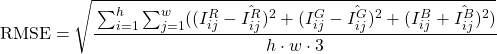where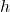— image height,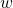— image width,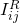— the intensity value for the pixel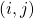of the panorama image generated in the red channel (— green channel,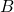— blue channel),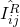— the intensity value for the pixelof the original image in the red channel (— green channel,— blue channel).

Figure 10 — The panorama image before graph adjustment (RMSE=35.3) and after (RMSE=14.2)

A graphic representation of RMSE on the test set looks as follows (see Figure 11):

Figure 11 — RMSE on the material of the test set. The images were sorted in increasing order of RMSE before graph adjustment was performed.

For each value of root mean square error before adjustment there was presented a value of root mean square error after graph adjustment. The median value of RMSE on the test set before graph adjustment is 33.5, after graph adjustment — 13.9.

Conclusion

Judging from the comparison results of the stitching quality, we can make a conclusion that graph adjustment considerably reduces cumulative error and improves the quality of panorama image stitching. However, we have to remember that graph adjustment will work only if there are cycles in the projective transformations graph. If there are no cycles present in the projective transformations graph, the graph adjustment module won’t have a deteriorating effect.

It is worth mentioning that this graph adjustment method works with a set of projective transformations and the method used to find these projective transformations is irrelevant here.

We are planning on optimizing the operating complexity of the algorithm in the future since it can be applied only to offline use-cases right now.

Bibliography

 Губин А.Ю., Ковин Р.В. Простой подход к задаче склейки перекрывающихся изображений в панораму // X Международная научно-практическая конференция студентов, аспирантов и молодых ученых «Молодежь и современные информационные технологии», с. 79-81, 2012.
 Drummond T., Rosten E. Machine Learning for high-speed corner detection // 9th European Conference on Computer Vision (ECCV), p. 430-443, 2006.
 Lowe D.G. Distinctive Image Features from Scale-Invariant Keypoints // International Journal of Computer Vision, p. 91-110, 2004.
 Bay H., Ess A., Yuitelaars T., Van Gool L. SURF: Speeded up robust features // Computer Vision and Image Understanding, v. 110, p. 346-359, 2008.
 Martin A. Fischler, Robert C. Bolles. Random sample consensus: A paradigm for model fitting with applications to image analysis and automated cartography // Comm. of the ACM, v. 24, p. 381-395, 1981.
 Арлазаров В.Л., Булатов К.Б., Чернов Т.С. Метод нечеткого поиска изображений в больших объемах видеоданных // Системы высокой доступности, Т. 12, № 1, с. 53-58, 2016.
 Skoryukina N. et al. Snapscreen: TV-stream frame search with projectively distorted and noisy query // 9th International COnference on Machine Vision (ICMV) — Proc. SPIE V. 10341, P. 103410Y, 2017.
 Bouguet J.Y. Pyramidal implementation of the affine lucas kanade feature tracker: destription of the algorithm // Intel corporation, V. 5, p. 1-10, 2001.
 Newman P., Ho K. SLAM-loop closing with visually salient features // IEEE Proc. of International Conference on Robotics and Automation, p. 635-642, 2005.
 Paalanen P., Kamarainen J.K., Kalviainen H. Image based quantitative mosaic evaluation with artificial video // Scandinavian Conference on Image Analysis, Springer (Berlin, Heidelberg), p. 470-479, 2009.